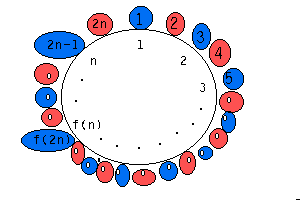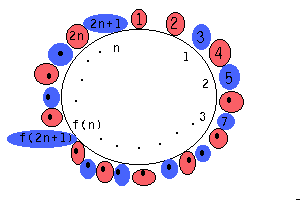The Josephus Problem and Recursion

Historian Flavius Josephus (37-93+AD) was a controversial writer/historian who chronicled the onslaught of the Romans against the Jewish people of his time.
One story attributed to him by Arthur Engle, though I haven't authenticate it, has him leading a group of 40 jews who, caught in a cave by the Romans, choose death over slavery.  As the story goes, it was decided (by Josephus?) that the 40 would line up (hap hazardly?) in a circle at starting at some (random?) point and counting around the circle, every seventh person would be killed, shortening the circle as they progressed.  It was agreed that the last survivor would commit suicide.  As the chronicler of the story (Josephus) has it, the only survivor was (by coincidence?) Josephus.  Josephus decided to "live to fight again," and enter the servitude of the Roman conqueror Titus, instead.

Did Josephus actually experience this? - and if he did, did he solve the problem of the last survivor beforehand?  In what follows we examine the Josephus problem and discover how to find

fk(n) = the number of the survivor of this process
with n original contestants eliminated in multiples of k.

I have written a program for the TI-85 which returns the number of the survivor,  fk(n).  You can read an annotated version of it here.  The program is inefficient and the reader is welcome to improve upon it.

The Simple Case Where k = 2.

The TI program I wrote is pretty blunt.  It creates a list of n complex numbers whose real part indicates their position in the circle and whose imaginary part is 0 if they're alive and 1 if they're dead. It then steps around the circle killing every kth live object until all but one are dead.

While there are many improvements that can be made to my TI program, TI basic is not able to come close to the efficiency of the following Pascal program found in Engel's book:

program jos;
var n : integer;
function  f(n : integer) : integer;         // declare function and variable.

begin
if n<3 then f := 1            // if n is 1 or 2, the survivor is 1
else if odd(n) then        // if n is odd, then the survivor on the inner circle is
f := 2*f(n div 2)+1     // whereas, if n is even then the survivor is
else f := 2*f(n div 2)-1   // Note that "n div 2" is the integer part of n/2.
end;

begin
write('n='); readln(n);     // This is the main part of the program
end.

Let's see how recursion works in this program.  Firstly, note that the program is recursive because the function f is defined in terms of itself.  A function call, f(12), in the main part of the program generates a computational process consisting of a series of expansions and contractions.  The expansion is the deferred multiplication by 2 together with either adding or subtracting 1 depending on whether the input to f is even or odd, while the contraction occurs when these operations are actually performed:

f(12) = 2*f(6)-1 = 2*(2*f(3)-1)-1 = 2*(2*(2*f(1)+1)-1)-1   (expansion)
=  2*(2*(2*1+1)-1)-1 =  2*(2*(3)-1)-1 =  2*(5)-1 = 9  (contraction)

Let's justify that this recursive scheme gives the solution to the .  And . . .rather than people being killed; which has become a tiresome premise, let's assume we're talking about blue balls turning red when their numbers come up.
If there is an even number, 2n, of blue balls in the circle and all the even numbered balls turn red, after which we renumber the ducks:  then duck number 2n - 1 is renumbered duck n.  Thus the original number of the survivor f(n) on the smaller circle was numbered  f(2n) = 2f(n) - 1 (see the picture on the left below.)
If there is an odd number, 2n+1, of blue balls in the circle and every other one turns red (again, the even-numbered ones - but this time duck number 1 also flies away,) after which the remaining ducks a renumbered -  then the original number of the survivor f(n) on the smaller, inner circle is f(2n+1) = 2f(n)+1.All of this suggests that, even though the TI-Basic language doesn't accomodate the slick recursion features of Turbo Pascal 7.0 from Borland, we could simulate the process by dividing n by 2 and putting a 1 or -1 into a list, depending on whether the resulting number is even or odd (expansion) and then using the list to construct f(n) by starting with 1 and doubling  with an addition or subtraction of 1 a number of times (contraction.)

The General Case Where k>1

Engel gives the the following Pascal code for an algorithm which produces the number, x, of the person who survives the (i-1)-st round, but not the i-th  round:

program joseph1;
var k, n, i, x : real;

begin
write('n,k,i=');
x := k*i;
while x>n do
x := int((k*(x-n)-1)/(k-1));
writeln('the ', i : 0 : 0, '-th elim. person has #', x : 0 : 0);
end.

Note that there is no function calling itself; instead, x is initialized as k*i and iterated according to the scheme
x := int((k*(x-n)-1)/(k-1)).
Let's see how this formula comes about.
Define the j-th count of a ball to be
xj  the count (without renumbering) the ball will have if the blue balls
are counted around and every ball numbered a multiple of k is turned red.

So if there are n = 5 blue balls originally and every third blue ball is turned red (k = 3) then the fourth ball survives and will have the sequence {x1=4, x2=8, x3=11} while the sequence for the first ball is {1, 6}

If  the ball  x1  has not turned red on round  j+1, then
(1)                xj+1 = xj + n - floor(xj/k).
Here "floor" means "the greatest integer less than."  To see that this is so, observe that the number of  red balls on the j-th round is floor(xj/k), so the number remaining is n - floor(xj/k) and adding this number to the previous count gives the next count.
Now  xj/k = floor(xj/k) + r/k, where the remainder, 1/k<= r/k<= (k-1)/k.  Note that the remainder can't be 0 or the ball would be turned red.  Solving for floor(xj/k) and substituting into equation (1) we get

xj+1 = xj + n - xj/k + r/k = n + r/k + xj(1 - 1/k)
<=>       xj = (xj+1 - n - r/k)*k/(k-1) = (xj+1 - n)*k/(k-1) - r/(k-1)

If this last term were sure to be less than 1 we could take the integer part of the first term and thus have a formula we could follow backwards to find the original x1 , but if  r=k-1  this doesn't work  So borrow 1/(k-1) from the first term:

xj =  [(xj+1 - n)*k - 1]/(k-1) - (r-1)/(k-1) = floor( [(xj+1 - n)*k - 1]/(k-1) )

Or, by subtracting and adding  (xj+1 - n)/(k-1), we have

xj =  floor( [(xj+1 - n)*(k-1) + xj+1 - n - 1]/(k-1) )   and finally,

(2)         xj = xj+1 - n + floor( (xj+1-n-1)/(k-1) )

Now, if xj  is divisible by k then the ball turns red and the sequence {xj} terminates.  At that point the ball becomes the (xj/k)-th red ball, thus the last count of the i-th red ball is xj = i*k.  So we start with this value and iterate back to the original x using (2).

Conclusion: With a little analytical thinking, what could be a long, difficult, arduous job is reduced to a trifle.

If you have comments or suggestions, email me.